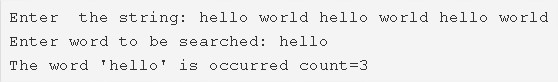# C Program To Count Occurrences Of A Word In A Given String | C Programs

C program to count the occurrences of a word in a given string – In this article, we will brief in on the multiple methods to count the occurrences of a word in a given string in C programming.

Suitable examples and sample programs have also been added so that you can understand the whole thing very clearly. The compiler has also been added with which you can execute it yourself.

The methods used in this blog are as follows:

• Using Standard Method
• Using Function

A string is nothing but an array of characters. The value of a string is determined by the terminating character. Its value is considered to be 0.Firstly, as given in the image above, you need to enter the string that you want to.

The string entered here is as follows:

“hello world hello world hello world”

Then, stipulate the world that you want to search in the string.

The word mentioned here is “hello”

The word “hello” occurs thrice in the whole string.

Thus, doing the same is C programming is as follows:

## Using Standard Method

1. Read the entered string and store the string in the variable ‘s’ using the function gets(s).

2) Read the entered word to be searched, store the word in the variable ‘w’ using gets(w) function.

3) The first for loop iterates through the string and place the locations of the white space of the string in the integer string a[].

4) j=0,The for loop iterates through the white spaces of the string with the structure for(i=0;i<k;i++)

a) Initialize n=index of the whitespace a[i]-j.

b) if n=length of the word then assign t=0 and compare the elements of the word with the elements of the string as s[l+j]==w[l] by increasing l value till the last element of the word. If the element matched then increase the t value.

If t=length of the word then increase the found value. Found indicates the number of occurrences of the word.

c) Initialize j=a[i]+1 to find the next occurrence of the word.

5) Print the total occurrences of the word in the string is the value of found.

Output:

### Using Function

1. The main() calls the check(char *s, char *w) function to find the total number of occurrences of the word in the string.

2) The function check()

a) The first for loop iterates through the string and stores the index values of the white spaces of the string in the integer array a[].

b) j=0,the 2nd for loop iterates through the white spaces of the string with the structure for(i=0;i<k;i++) and initialize n=index of the whitespace a[i]-j.

b.1) If n=length of the word then compare s[l+j] with w[l] using for loop for(l=0;w[l];l++). If they matched then increase the t value.

b.2) If t=length of the word then increase the found value. i.e for each occurrence of the word the found value will be increased.

b.3) Initialize j=a[i]+1.

The function returns the found value. Then the main() prints the found value i.e the total number of occurrences of the word in the string.

Output:

x

## C Program To Check If Alphabet, Digit or Special Character | C Programs

C program to check for an alphabet, digit or special character – In this article, ...